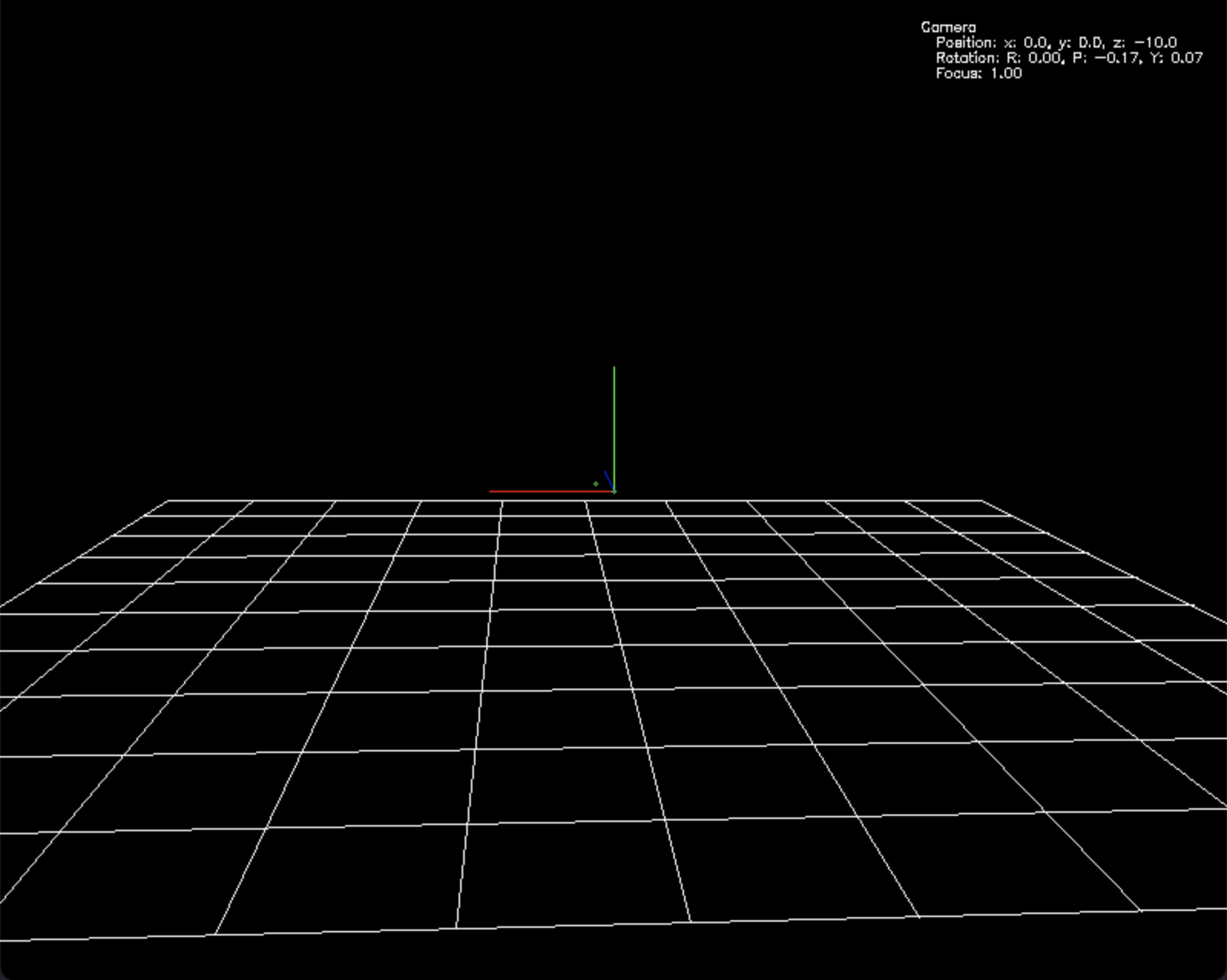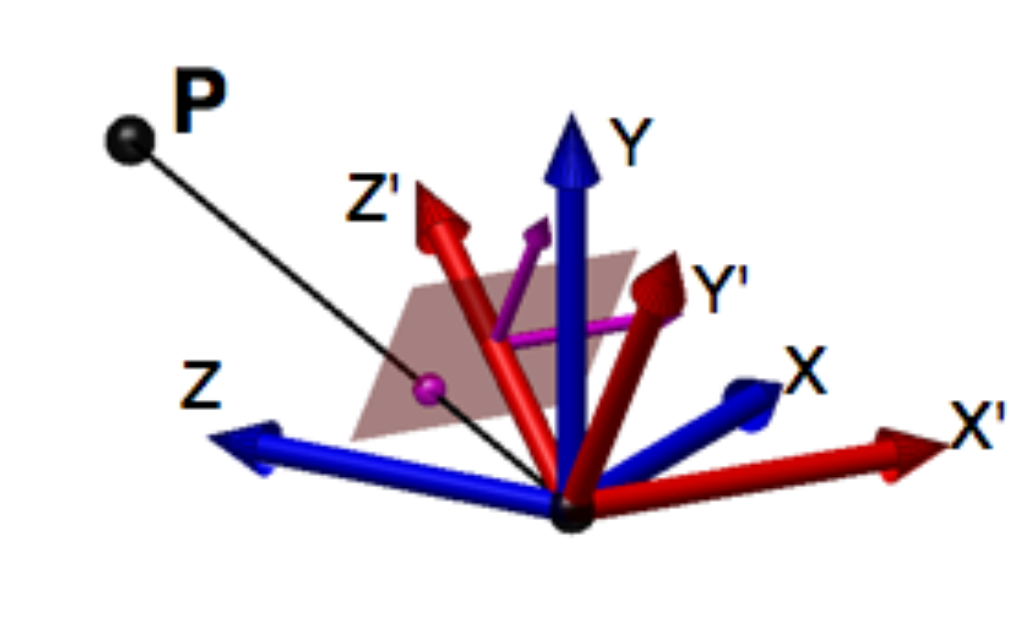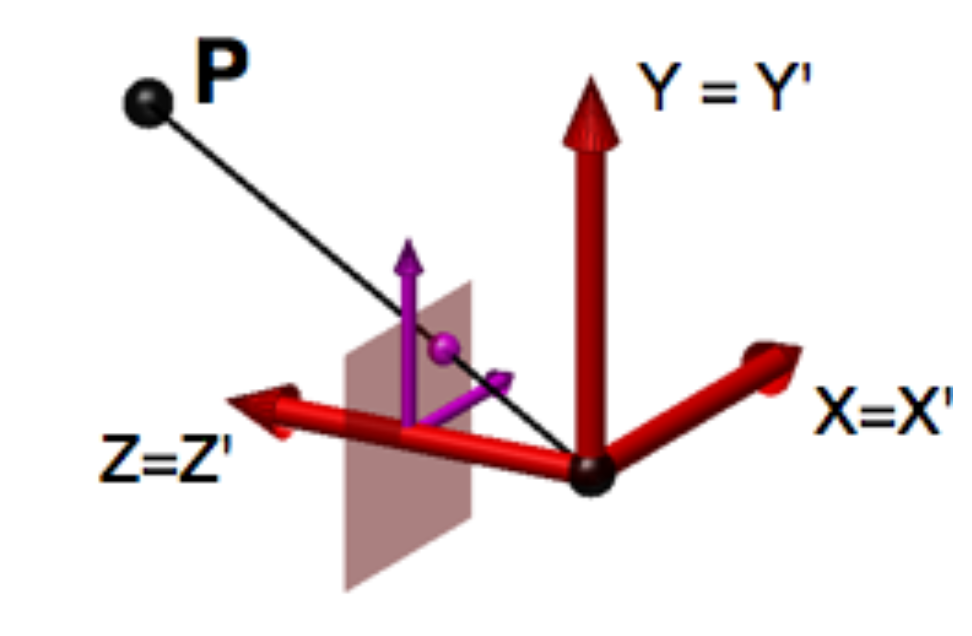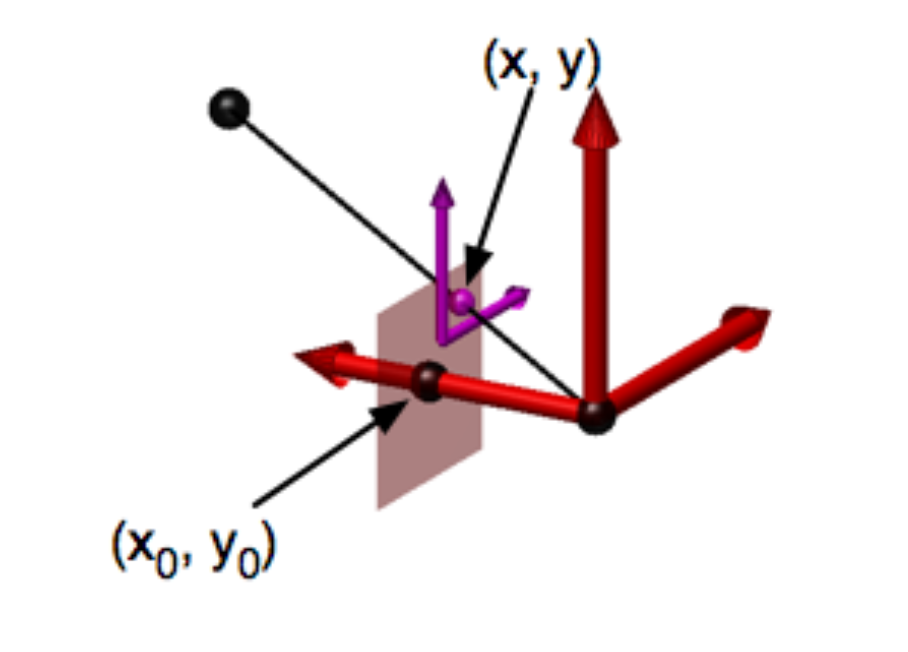# 目录

3D 设计软件和游戏中的图像经常是以一个观测者的角度展示的，可以把这个过程想象成一个人拿着一台摄像机在拍摄，在机器视觉中叫摄像机模型。# 摄像机模型的数学模型

## 座标系

• 世界座标系 (World frame)

被观测目标存在于世界座标系中，使用世界座标系座标表示位置，是个 3 维座标系，可以使用常用的单位，比如‘米’。

• 相机座标系 (Camera frame)

通常使用摄像机的光学中心为原点，是一个 3 维座标系，表示从相机的光学中心原点来衡量各个目标的位置，尺度可以保持和世界座标系统一，比如都使用‘米’；相机可以移动到世界座标系中的任意位置和任意角度（姿态）。

• 像平面座标系 (Image plane frame)

这是最终观测的图像座标系，是一个 3 维座标系，在相机中是 CCD 的座标系，例如以左上角为原点，尺度为‘像素’。## 座标转换

1. 把世界座标系中的物体座标进行’座标系变换’，转换成相机座标系中的座标
2. 通过相机内参转换到像平面座标系

### 座标系变换过程

$$\left( \begin{matrix} X_w Y_w Z_w \end{matrix} \right)$$

$$\left( \begin{matrix} x_t y_t z_t \end{matrix} \right)$$

$$\left( \begin{matrix} O_w - t \end{matrix} \right)= \left( \begin{matrix} X_w - t_x \\\ Y_w - t_y \\\ Z_w - t_z \end{matrix} \right)$$$$O_c = R* \left( O_w - t \right)= R* \left( \begin{matrix} X_w - t_x \\\ Y_w - t_y \\\ Z_w - t_z \end{matrix} \right) = \left( \begin{matrix} X_c \\\ Y_c \\\ Z_c \end{matrix} \right)$$### 相机座标转化到像平面座标$$x‘ = f \frac {X_c} {Z_c}$$ $$y’ = f \frac {Y_c} {Z_c}$$ $$z‘ = f \frac {Z_c} {Z_c}$$

$$\left( \begin{matrix} x' \\\ y' \\\ f \end{matrix} \right)= f/Z \left( \begin{matrix} X_c \\\ Y_c \\\ Z_c \end{matrix} \right)$$

$$\left( \begin{matrix} x' \\\ y' \\\ f \end{matrix} \right)= \left( \begin{matrix} \frac {x - x_o} {\sigma_x} \\\ \frac {y - x_o} {\sigma_y} \\\ f \end{matrix} \right)= f/Z \left( \begin{matrix} X_c \\\ Y_c \\\ Z_c \end{matrix} \right)$$

$$\left( \begin{matrix} \frac {x - x_o} {\sigma_x} \\\ \frac {y - x_o} {\sigma_y} \\\ f \end{matrix} \right)= \left( \begin{matrix} \frac 1 {\sigma_x} & 0 & 0 \\\ 0 & \frac 1 {\sigma_y} & 0 \\\ 0 & 0 & 1 \end{matrix} \right) \left( \begin{matrix} x - x_o \\\ y - y_o \\\ f \end{matrix} \right)= f/Z \left( \begin{matrix} X_c \\\ Y_c \\\ Z_c \end{matrix} \right)$$

$$\left( \begin{matrix} x - x_o \\\ y - y_o \\\ f \end{matrix} \right)= f/Z \left( \begin{matrix} \sigma_x & 0 & 0 \\\ 0 & \sigma_y & 0 \\\ 0 & 0 & 1 \end{matrix} \right) \left( \begin{matrix} X_c \\\ Y_c \\\ Z_c \end{matrix} \right)$$

$$\left( \begin{matrix} x - x_o \\\ y - y_o \\\ f \end{matrix} \right)= \left( \begin{matrix} 1 & 0 & -\frac {x_o} f \\\ 0 & 1 & -\frac {y_o} f \\\ 0 & 0 & 1 \end{matrix} \right) \left( \begin{matrix} x \\\ y \\\ f \end{matrix} \right)= f/Z \left( \begin{matrix} \sigma_x & 0 & 0 \\\ 0 & \sigma_y & 0 \\\ 0 & 0 & 1 \end{matrix} \right) \left( \begin{matrix} X_c \\\ Y_c \\\ Z_c \end{matrix} \right)$$

$$\left( \begin{matrix} x \\\ y \\\ f \end{matrix} \right)= f/Z \left( \begin{matrix} 1 & 0 & \frac {x_o} f \\\ 0 & 1 & \frac {y_o} f \\\ 0 & 0 & 1 \end{matrix} \right) \left( \begin{matrix} \sigma_x & 0 & 0 \\\ 0 & \sigma_y & 0 \\\ 0 & 0 & 1 \end{matrix} \right) \left( \begin{matrix} X_c \\\ Y_c \\\ Z_c \end{matrix} \right)= 1/Z \left( \begin{matrix} f \sigma_x & 0 & x_o \\\ 0 & f \sigma_y & y_o \\\ 0 & 0 & 1 \end{matrix} \right) \left( \begin{matrix} X_c \\\ Y_c \\\ Z_c \end{matrix} \right)$$

## 摄像机数学模型

$$O_c = R* \left( O_w - t \right)= R* \left( \begin{matrix} X_w - t_x \\\ Y_w - t_y \\\ Z_w - t_z \end{matrix} \right) = \left( \begin{matrix} X_c \\\ Y_c \\\ Z_c \end{matrix} \right)$$

$$\left( \begin{matrix} x \\\ y \\\ f \end{matrix} \right)= 1/Z \left( \begin{matrix} f \sigma_x & 0 & x_o \\\ 0 & f \sigma_y & y_o \\\ 0 & 0 & 1 \end{matrix} \right) \left( \begin{matrix} X_c \\\ Y_c \\\ Z_c \end{matrix} \right)$$

# 实现一个摄像机模型

      def rotate(self, roll, pitch, yaw):
'''
Rotate camera by roll, pitch, yaw
'''
rx, _ = cv2.Rodrigues((pitch, 0, 0))
Rodrigues(src, dst=None, jacobian=None, /) -> dst, jacobian


      def trans_to_cam(self, v):
'''
Transform the world coordinate vertices to camera coordinate vertices
v: vertices in world coordinate frame
'''
vc = np.dot(self.R, (v.T - np.array([[self._x], [self._y], [self._z]])))
return vc.T


          # camera focus length in meter
self._f = 1

# scale factor: pixels/meter
self._s = 800

# camera intrinsic matrix
self.intrinsic = np.array([[self._f*self._s,    0,                  self._canvas_width/2.0],
[0,                  self._f*self._s,    self._canvas_height/2.0],
[0,                  0,                  1]])


      def project(self, v):
'''
Project the vertices of camera coordinate to camera image plane coordinate
v: vertices in camera coordinate frame
u = width - f*(Y/X)
v = height - f*(Z/X)
'''
Z = np.expand_dims(v[:, -1], axis=1)
proj_v = np.dot(self.intrinsic, v.T) / Z
proj_v = proj_v.T
proj_v[:, 0:2] = [self._canvas_width, self._canvas_height] - proj_v[:, 0:2]
return proj_v[:, :2]


Demo：